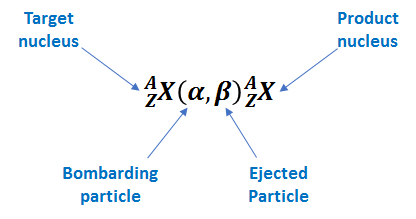# Problem: Each of the following transmutations produces a radionuclide used in positron emission tomography (PET).(i) 14N(p , α)X (ii) 18O(p, X)18F (iii) 14N(d, n)15OIn equation (i), identify the species signified as "X".

###### FREE Expert Solution

We’re being asked to identify the species signified as X in the given nuclear transmutation reactions.

Nuclear transmutation is changing one nuclide (chemical element) or an isotope into another chemical element.

A shorthand notation is used to represent nuclear transmutations lists the target nucleus, the bombarding particle and the ejected particle in parentheses, then the product nucleus.Given: 14N(p,α)X

97% (378 ratings)###### Problem Details

Each of the following transmutations produces a radionuclide used in positron emission tomography (PET).
(i) 14N(p , α)X
(ii) 18O(p, X)18F
(iii) 14N(d, n)15O

In equation (i), identify the species signified as "X".